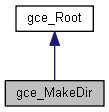# gce_MakeDir Class Reference

This class implements the following algorithms used to create a Dir from gp. More...

`#include <gce_MakeDir.hxx>`

Inheritance diagram for gce_MakeDir:[legend]

## Public Member Functions

gce_MakeDir (const gp_Vec &V)
Normalizes the vector V and creates a direction. Status is "NullVector" if V.Magnitude() <= Resolution. More...

gce_MakeDir (const gp_XYZ &Coord)
Creates a direction from a triplet of coordinates. Status is "NullVector" if Coord.Modulus() <= Resolution from gp. More...

gce_MakeDir (const Standard_Real Xv, const Standard_Real Yv, const Standard_Real Zv)
Creates a direction with its 3 cartesian coordinates. Status is "NullVector" if Sqrt(Xv*Xv + Yv*Yv + Zv*Zv) <= Resolution. More...

gce_MakeDir (const gp_Pnt &P1, const gp_Pnt &P2)
Make a Dir from gp <TheDir> passing through 2 Pnt <P1>,<P2>. Status is "ConfusedPoints" if <p1> and <P2> are confused. Warning If an error occurs (that is, when IsDone returns false), the Status function returns: More...

const gp_DirValue () const
Returns the constructed unit vector. Exceptions StdFail_NotDone if no unit vector is constructed. More...

const gp_DirOperator () const

operator gp_Dir () constPublic Member Functions inherited from gce_Root
Standard_Boolean IsDone () const
Returns true if the construction is successful. More...

gce_ErrorType Status () const
Returns the status of the construction: More...Protected Attributes inherited from gce_Root
gce_ErrorType TheError

## Detailed Description

This class implements the following algorithms used to create a Dir from gp.

• Create a Dir parallel to another and passing through a point.
• Create a Dir passing through 2 points.
• Create a Dir from its axis (Ax1 from gp).
• Create a Dir from a point and a direction.

## Constructor & Destructor Documentation

 gce_MakeDir::gce_MakeDir ( const gp_Vec & V )

Normalizes the vector V and creates a direction. Status is "NullVector" if V.Magnitude() <= Resolution.

 gce_MakeDir::gce_MakeDir ( const gp_XYZ & Coord )

Creates a direction from a triplet of coordinates. Status is "NullVector" if Coord.Modulus() <= Resolution from gp.

 gce_MakeDir::gce_MakeDir ( const Standard_Real Xv, const Standard_Real Yv, const Standard_Real Zv )

Creates a direction with its 3 cartesian coordinates. Status is "NullVector" if Sqrt(Xv*Xv + Yv*Yv + Zv*Zv) <= Resolution.

 gce_MakeDir::gce_MakeDir ( const gp_Pnt & P1, const gp_Pnt & P2 )

Make a Dir from gp <TheDir> passing through 2 Pnt <P1>,<P2>. Status is "ConfusedPoints" if <p1> and <P2> are confused. Warning If an error occurs (that is, when IsDone returns false), the Status function returns:

• gce_ConfusedPoints if points P1 and P2 are coincident, or
• gce_NullVector if one of the following is less than or equal to gp::Resolution():
• the magnitude of vector V,
• the modulus of Coord,
• Sqrt(Xv*Xv + Yv*Yv + Zv*Zv).

## Member Function Documentation

 const gp_Dir& gce_MakeDir::Operator ( ) const
 gce_MakeDir::operator gp_Dir ( ) const
 const gp_Dir& gce_MakeDir::Value ( ) const

Returns the constructed unit vector. Exceptions StdFail_NotDone if no unit vector is constructed.

The documentation for this class was generated from the following file: